Courses

# Effect of Damping Notes | EduRev

## Mechanical Engineering : Effect of Damping Notes | EduRev

The document Effect of Damping Notes | EduRev is a part of the Mechanical Engineering Course Theory of Machines (TOM).
All you need of Mechanical Engineering at this link: Mechanical Engineering

Damped Systems

• Damping is an effect which causes a Reduction in the amplitude of an oscillation as a Result of energy being drained from the system to overcame frictional/other Resistive forces.
• These are the systems in which kinetic friction is zero. Technical Name of Kinetic friction in any Vibrations system is known as, Damping.

Damping in Any System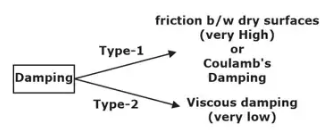Damping force ox = (x = velocity)
= c·x
Where, c = coefficient of damping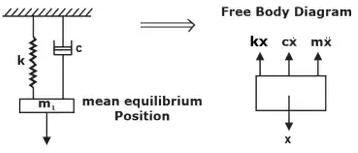The equilibrium equation of a damped spring-mass system is given as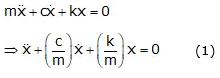It is a 2nd order differential equation.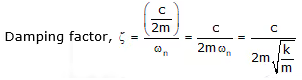Types of Damping Systems

1. Overdamped System(ζ>1)
No vibrations will be present in an over-damped system.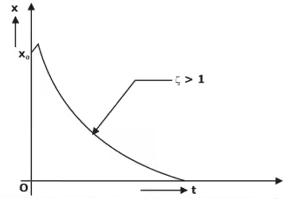Critically Damped Systems(ζ = 1):
∴ No Vibrations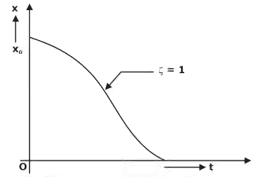Note: Critical damping response is much faster than overdamping response i.e. in critically-damped systems, the body once displaced comes into its Equilibrium Position much faster than that in overdamped systems.
2. Under Damped Systems (ζ < 1)
∴ Damped frequency, ωd = ωn(1 - ξ2)1/2
Time period Td = 2π/wd (Second)
Linear frequency, fn = 1/Td (Hz)
Hence, Amplitude of under-Damped Vibrations is not constant and it decreases exponentially w.r.t. time.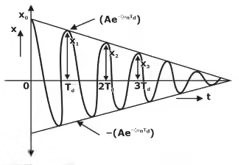3. Logarithmic Decrement (δ)
δ = In (Decrement ratio)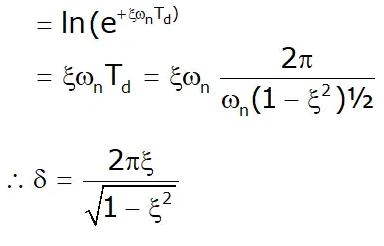Offer running on EduRev: Apply code STAYHOME200 to get INR 200 off on our premium plan EduRev Infinity!

## Theory of Machines (TOM)

94 videos|41 docs|28 tests

,

,

,

,

,

,

,

,

,

,

,

,

,

,

,

,

,

,

,

,

,

;